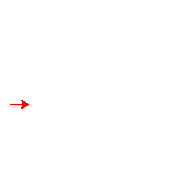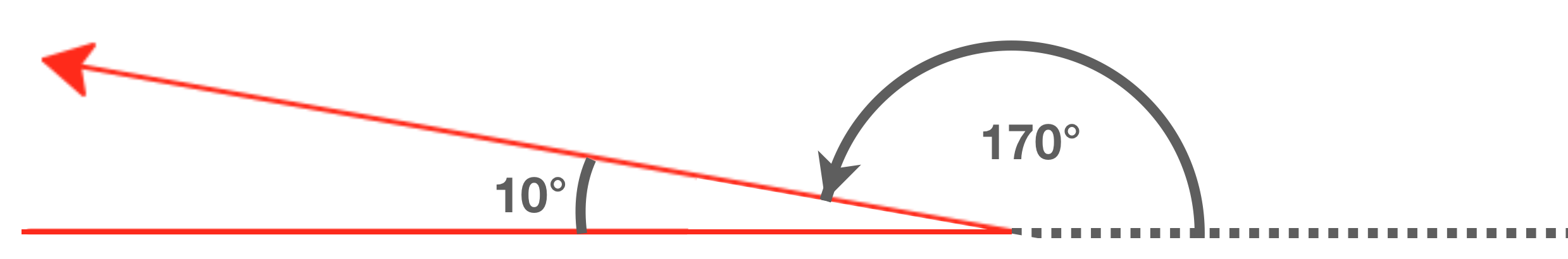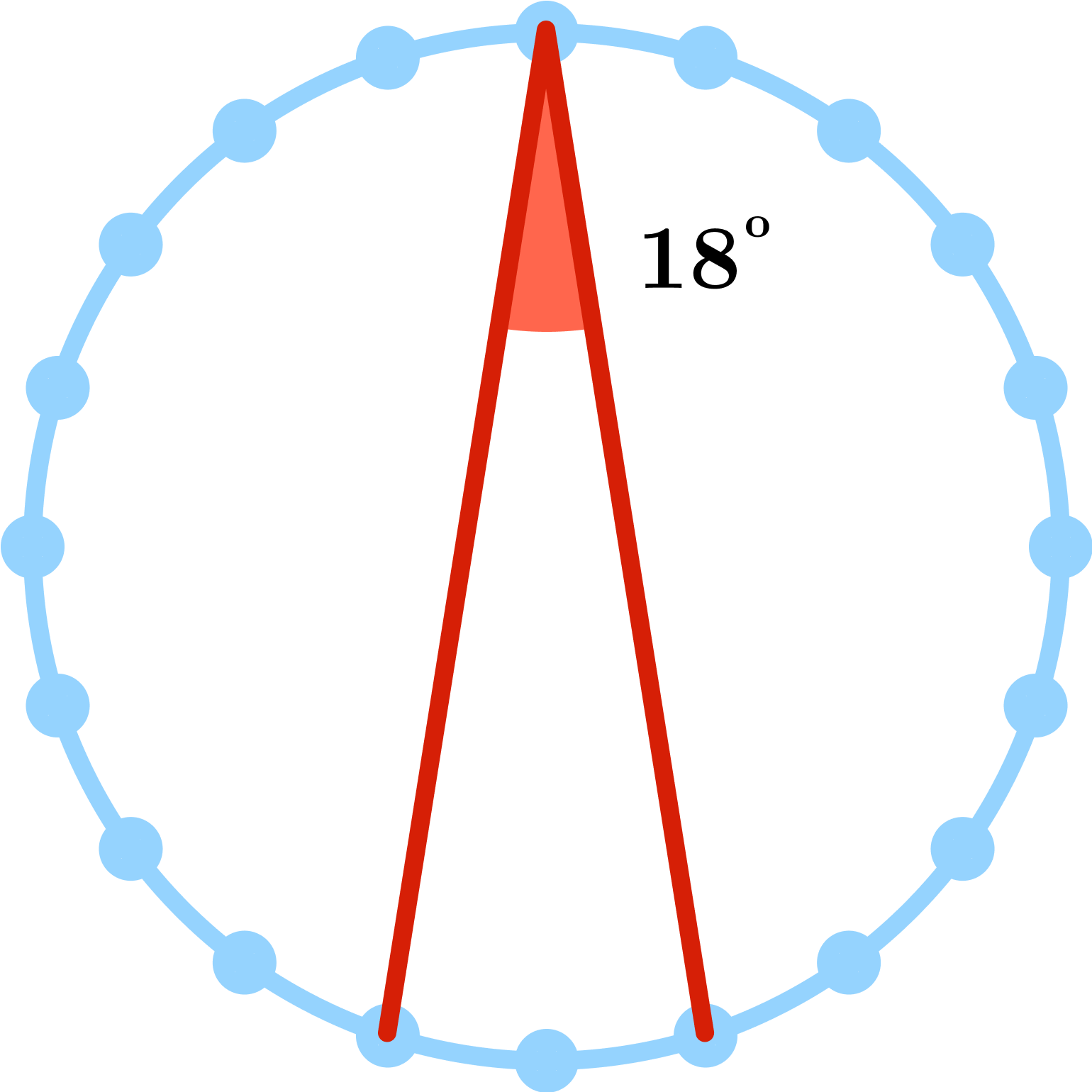Programming with Python

Many programming courses start by teaching how to create programs that execute mathematical calculations. While applications to calculations are the historical origins of computer programming, and we'll see plenty of that eventually, we want to introduce you to Python, and programming in general, in a more creative context: drawing.Drawing Many-Pointed Stars

The code below is deceptive! In addition to being deceptive, it includes a for loop, which still hasn't been fully explained.

Don't worry about understanding this (deceptive, unfamiliar) code yet. Just run it to see what it does!

What does it draw?

from turtle import *
color('red')

for i in range(20):
forward(200)
left(108)

bye()
Python 3
You need to be connected to run code

Drawing Many-Pointed Stars

Here is a less deceptive version of the code from before. The for instruction causes instructions to be run multiple times, and right now you don't need to know what i in range means. The number 10 means that indented lines inside the loop will repeat 10 times.

from turtle import *
color('red')

for i in range(10):
left(108)
forward(200)

bye()

This is the start of a for-loop. Don't worry yet what 'i' and 'range' mean here. Just know that what follows will be repeated ('looped over') 10 times.

The indentation marks that we are now inside the loop. In Python, all the indented lines that follow the line for i in range(10) will be repeated 10 times.

This line is also indented. We run the entire indented segment 10 times, so we will turn left and then move forward 10 times. (We won't turn left 10 times and then move forward 10 times.)

No more indentation! This marks the end of the for-loop.

You need to be connected to run code

Now you're ready to understand the code inside the loop.

Drawing Many-Pointed Stars

Before you looked at the 10-pointed star, all the code you'd seen only turned 90 degrees left or right.

For drawing stars, you need more careful control to specify exactly how far the turtle moves as it draws and how much it turns.

• The command left(108) will make the turtle turn (on the spot) 108 degrees to the left (counterclockwise). Any number can be put in the parentheses: left(60) would make the turtle turn left 60 degrees, and left(170) would make the turtle turn left 170 degrees.
• Similarly, right(60) makes it turn 60 degrees to the right
• The command forward(150) will make the turtle move 150 pixels forward in the direction it is presently facing. forward(90) will make the turtle move 90 pixels forward.

One important, unintuitive note:

The turning angle inside the left (and right) commands is not the interior angle of the turn. Rather, it is how much the turtle turns from the direction it had previously been facing.So to make this really sharp turn, you use the command left(170).

Drawing Many-Pointed Stars

Try to figure this one out before you hit "Run code," then run the code to check your answer.

What will this program draw?

from turtle import *
color('red')

for i in range(6):
forward(300)
left(120)

bye()
Python 3
You need to be connected to run code

Drawing Many-Pointed Stars

Drawing a 20-point star would take you quite a while with paper and pencil, but with a carefully constructed drawing program, you don't even have to list the steps in the code 20 times! Instead, this little program uses a loop to repeat two instructions over and over:

1. forward(200) means "move forward 200 pixels."
2. left(216) means "turn counterclockwise 216 degrees."

And the line just before these directions, for i in range(20):, is the line that controls the loop and specifies how many times the immediately following instructions get repeated: 20 times.

Run the code below and then change the 20 to 5 so that the instructions to move forward and turn 216 degrees only repeat five times instead of 20. What's the difference between the star that's drawn in each case?

from turtle import *
color('red')

for i in range(20):
forward(200)
left(216)

bye()
Python 3
You need to be connected to run code

Drawing Many-Pointed StarsHere is the same program again. Your task now is to figure out how to set the three numbers below to make a 20-point star.

Hint: If you evenly space 20 points around a circle, zig-zagging from one side of the circle to a point almost, but not-exactly across and then back again makes an 18 degree angle.

Of the options shown, what is the smallest positive value that you can set the left turning angle to if your goal is to draw a 20-point star?

from turtle import *
color('red')

for i in range(20):
forward(200)
left(216)

bye()
Python 3
You need to be connected to run code

Drawing Many-Pointed Stars

Now how could we change the color of the star? E. g. make it purple, or green? Which line could you edit to change the star's coloring?

from turtle import *
color('red')

for i in range(20):
forward(200)
left(216)

bye()
Python 3
You need to be connected to run code

Drawing Many-Pointed Stars

This course will cover loops, nested loops, conditionals, and recursion! While the structure of a single loop may be simple, learning how to plan out and manage the control-flow of your programs is one of the toughest parts of programming.

That's all for now, but you're welcome to explore this star-drawing program a bit more on your own before continuing!

Challenge 1: What happens if you alternate between two different edge lengths?
(Run the code below to see.)
Challenge 2: What happens if you alternate between two different turn angles?
Challenge 3: Can you make a 12-point star that's perfectly symmetric?

Loops can execute any number of instructions, just make sure that each instruction is on its own line and indented once inwards from the loop header, for i in range(5):.

from turtle import *
color('red')

for i in range(5):
forward(200)
left(216)
forward(150)
left(216)

bye()
Python 3
You need to be connected to run code

Drawing Many-Pointed Stars

×